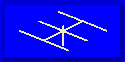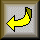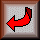## Voltage, Current, and ImpedanceAlong a Transmission Line

### L. B. Cebik, W4RNLA few years ago, I made up a little utility program in GW BASIC to satisfy my curiosity about--and later my need to know--what happens to voltage, current, and impedance along a transmission line. The program used lossless lines, since I was interested in identifying the recurring and nonrecurring values along the line. If someone needs to know the values with line losses factored in, N6BV has an excellent program on the ARRL bulletin board.

What I wanted was less a specific value for a predetermined cut of line (although the program figures that, too), but a table of values about every 5 degrees to show me how and at what rates the voltage, current, and impedance were changing under various matches and mismatches between the antenna and transmission line.

I wanted not only to examine the impedance--our obsession with which has fouled up many an antenna analysis--but as well to observe the changes in voltage and current, along with their phase angles. These latter figures are essential to understanding phase lines and other matters of matching. For example, in a ZL Special, it is crucial to set up the elements and the phasing line so that the rear element sees a current magnitude and phase that is correct relative to the values on the forward element, which also yields a certain value of voltage at the feedpoint common to both element feed systems. Although the characteristic impedance of the line plays a critical role in the transformation, the actual element impedances are secondary.

Because someone else may get interested in charting such data for lossless lines, the utility program is listed below. It is messy programming, because different parts of the data stream were added at different times. Impedance and phase are derived from values for R and +/-jX, which are separately calculated. Voltage and current, along with their phase angles, are redundantly calculated independently of each other and of impedance (just to see if I could get all the angle correction factors to work).

The program produces on screen 90 degrees of values. Hard copy produces 180 degrees--not much more will fit on a single piece of paper. To examine the other 180 degrees of a full cycle, simple make another run, plugging in the values for the 180-degree mark as the initial values.

Some of the values will surprise you. You may not realize how high voltages and currents can rise under seriously mismatched antenna-to-line conditions. You might not have known that, when the antenna exhibits a very high resistance and reactance, the line spends most of its length at very low values, with only brief rises to the heights. There is much to be learned from these tables.

A version of this program, smoothed in appearance by George Murphy, VE3ERP, is contained in HAMCALC, now in its 25th version. If you do not want to deal with this program directly in your own copy of BASIC, you can obtain a copy of the latest version of HAMCALC by sending a \$5 (US) donation to VE3ERP, 77 McKenzie Street, Orillia, Ontario L3V 6A6, Canada. Any funds beyond disks and postage, Murph sends to the Canadian National Institute for the Blind amateur radio program. HAMCALC is now up to over 140 utility calculation programs for almost everything a ham could ever want or need, short of antenna or propagation modeling. It has structured directories, an index, and its own copy of GW BASIC.

The other alternative is simply to clip off everything but the program from a downloaded ASCII version of this page. BASIC will read a program in ASCII, and you can then save it as a regular BASIC program. Replace the last line (1140) with your own favorite ending: I keep all my BASIC utility programs in a master menu for ease of finding and using them. In any event, have fun with the following morass of lines.```10 'FILE: "LINEIMP.BAS"
20 CLS:COLOR 11,1,3:CLS
30
IL=0:EL=0:THI=0:THE=0:THIR=0:THER=0:IIM=0:IRL=0:IPOL=0:IPHS=0:IPHSD=0:EIM=0
:ERM=0:EPOL=0:EPHS=0:EPHSD=0:RL=0:XL=0:ZO=0:VF=0:F=0:LD=0:LM=0:LF=0:LR=0:T=
0:TS=0:DA=0:DB=0:DN=0:RS=0:XS=0:XL=0:XM=0:XN=0:RZ=0:XZ=0:RI=0:XI=0:LN=0:LG=
0:RA=0:XA=0:LL=0
31 XXX\$=STRING\$(79,32)
40 PRINT"      Ein, Iin, Rin, and Xin for Line Lengths, given Antenna E, I,
R,and X"
50 PRINT "                              L. B. Cebik, W4RNL"
60 PRINT:PRINT "Calculates Input E, I, R, and X for specified transmission
lines, given the     'terminal' E, I, R, and X of the antenna.
Calculations can be for a specific   length of line (in degrees, feet, or
meters) or, more generally, for every five
70 PRINT "degrees of an unspecified line length (up to 90 degrees on screen
or 180 degreeson a print out).  Data can be from MININEC, NEC or
measurements.":PRINT
71 INPUT "Enter Antenna R in Ohms  ", RL
72 INPUT "Enter Antenna X in Ohms  ", XL
73 PRINT"Do you wish to enter (v)oltage and current or (p)ower?"
74 AA\$=INKEY\$:IF AA\$="v" OR AA\$="V" THEN 100 ELSE IF AA\$="P" OR AA\$="p"
THEN 75 ELSE 74
75 LOCATE 12,1:PRINT XXX\$:LOCATE 12,1:INPUT "Enter Load Power in Watts
",PWR
76
IL=SQR(PWR/RL):THI=0:EL=IL*SQR((RL^2)+(XL^2)):THER=ATN(XL/RL):PI=3.141593:T
HE=(THER*180)/PI
77 LOCATE 12,1:PRINT XXX\$:LOCATE 12,1:PRINT "Antenna Load Current in Amps
",IL
78 PRINT "Load Current Phase Angle in Degrees  ",THI
79 PRINT "Antenna Load Voltage in Volts  ",EL
80 PRINT "Load Voltage Phase Angle in Degrees  ",THE
81 GOTO 140
100 LOCATE 12,1:PRINT XXX\$:LOCATE 12,1:INPUT "Enter Antenna Load Current in
Amps (usually 1)  ",IL
110 INPUT "Enter Load Current Phase Angle in Degrees  ",THI
111 INPUT "Enter Antenna Load Voltage in Volts  ",EL
112 INPUT "Enter Load Voltage Phase Angle in Degrees  ",THE
113 PWR=IL*RL
140 INPUT "Enter Line Characteristic Impedance in Ohms  ", ZO
150 INPUT "Enter Line Velocity Factor as a decimal  ", VF
160 INPUT "Enter Frequency in MHz  ", F
170 INPUT "Do you wish to use a specific line length?  (Y)es or (N)o?  ",A\$
180 IF A\$="Y" OR A\$="y" THEN 200
190 IF A\$="N" OR A\$="n" THEN 500 ELSE 170
200 INPUT "Specify units for line length: (D)egrees, (F)eet, (M)eters  ",U\$
210 INPUT "Line Length  ",LL
220 IF U\$="D" OR U\$="d" THEN LD=LL
230 IF U\$="F" OR U\$="f" THEN LD=((.3660131*F)*LL)/VF
240 IF U\$="M" OR U\$="m" THEN LD=((1.200831*F)*LL)/VF
250 GOTO 750
260 INPUT "Do you wish a hard copy? (Y)es or (N)o? ",PR\$
270 IF PR\$="Y" OR PR\$="y" THEN PR=1 ELSE PR=0
280 CLS:PRINT "               Line Input R and X for Frequency ";F;"MHz"
290 IF PR=1 THEN LPRINT "               Line Input R and X for Frequency
";F;"MHz"
300 PRINT:PRINT "Ant I=";IL;"Amps at ";THI;"degrees;  Ant E=";EL;"Volts at
";THE;"degrees"
310 IF PR=1 THEN LPRINT:LPRINT "Ant I=";IL;"Amps at ";THI;"degrees;  Ant
E=";EL;"Volts at ";THE;"degrees"
320 PRINT "Ant R=";RL;"Ohms","   Ant X=";XL;"Ohms","  Power=";PWR;"Watts"
330 IF PR=1 THEN LPRINT "Ant R=";RL;"Ohms","   Ant X=";XL;"Ohms","
Power=";PWR;"Watts"
340 PRINT "Line Zo=";ZO;"Ohms","   Vel Fctr=";VF
350 IF PR=1 THEN LPRINT "Line Zo=";ZO;"Ohms","   Vel Fctr=";VF
360 PRINT:PRINT "    Line E      Phase     Line I    Phase    Line Z
Phase"
370 IF PR=1 THEN LPRINT:LPRINT "    Line E     Phase     Line I    Phase
Line Z     Phase"
380 PRINT USING "#######.##";EPOL,EPHSD,IPOL,IPHSD,Z,THZD
390 IF PR=1 THEN LPRINT USING "#######.##";EPOL,EPHSD,IPOL,IPHSD,Z,THZD
400 PRINT:PRINT "Degree","Feet","Meters","Rin","Xin"
410 IF PR=1 THEN LPRINT:LPRINT "Degree","Feet","Meters","Rin","Xin"
420 PRINT LD,LG,LN,:PRINT USING "#####.##";RI;:PRINT "     ";:PRINT USING
"#####.##";XI
430 IF PR=1 THEN LPRINT LD,LG,LN,:LPRINT USING "#####.##";RI;:LPRINT "
";:LPRINT USING "#####.##";XI:LPRINT:LPRINT
440 PRINT:PRINT:PR=0
450 INPUT "Would you like another run? (Y)es or (N)o?  ",R\$
460 IF R\$="Y" OR R\$="y" THEN GOTO 480
470 IF R\$="N" OR R\$="n" THEN 1140 ELSE 450
480 PRINT:PRINT "Press (n) to create a whole new entry; press (z) to begin
with a new line       characteristic impedance; press (l) to begin with a
new line length.":PRINT
490 I\$=INKEY\$:IF I\$="N" OR I\$="n" THEN GOTO 20 ELSE IF I\$="Z" OR I\$="z"
THEN GOTO 140 ELSE IF I\$="L" OR I\$="l" THEN GOTO 200 ELSE 490
500 INPUT "Do you wish a hard copy? (Y)es or (N)o? ",PR\$
510 IF PR\$="Y" OR PR\$="y" THEN PR=1 ELSE PR=0
520 CLS
530 PRINT "Values of I, E, Phase Angles, R, and X for Frequency ";F;"MHz"
540 IF PR=1 THEN LPRINT "Values of I, E, Phase Angles, R, and X for
Frequency ";F;"MHz"
550 PRINT:PRINT "Ant I=";IL;"Amps at ";THI;"degrees;  Ant E=";EL;"Volts at
";THE;"degrees"
560 IF PR=1 THEN LPRINT:LPRINT "Ant I=";IL;"Amps at ";THI;"degrees;  Ant
E=";EL;"Volts at ";THE;"degrees"
570 PRINT "Ant R=";RL;"Ohms","   Ant X=";XL;"Ohms";"  Power=";PWR;"Watts"
580 IF PR=1 THEN LPRINT "Ant R=";RL;"Ohms","   Ant X=";XL;"Ohms";"
Power=";PWR;"Watts"
590 PRINT "Line Zo=";ZO;"Ohms","   Vel Fctr=";VF,"Freq=";F;"MHz
600 IF PR=1 THEN LPRINT "Line Zo=";ZO;"Ohms","   Vel Fctr=";VF:LPRINT
610 PRINT "Degree   Feet     Ein      Phase   Iin     Phase    Rin      Xin
Z Phase   Z"
620 IF PR=1 THEN LPRINT "Degree   Feet     Ein      Phase   Iin     Phase
Rin      Xin   Z Phase   Z"
630 IF PR=1 THEN DE=180 ELSE DE=90
640 FOR LD=0 TO DE STEP 5
650 GOTO 750
660 PRINT USING "#####.##";LD,LG,EPOL,EPHSD,IPOL,IPHSD,RI,XI,THZD,Z
670 IF PR=1 THEN LPRINT USING
"#####.##";LD,LG,EPOL,EPHSD,IPOL,IPHSD,RI,XI,THZD,Z
680 NEXT
690 IF PR=1 THEN
LPRINT:LPRINT:LPRINT:LPRINT:LPRINT:LPRINT:LPRINT:LPRINT:LPRINT:LPRINT:LPRIN
T:LPRINT:LPRINT:LPRINT:LPRINT:LPRINT
700 INPUT "Do you wish another run? (Y)es or (N)o?  ",B\$
710 IF B\$="Y" OR B\$="y" THEN 730
720 IF B\$="N" OR B\$="n" THEN 1140 ELSE 700
730 PRINT:PRINT "Press (n) to create a whole new entry; or press (z) to
begin with a new line    characteristic impedance.  "
740 I\$=INKEY\$:IF I\$="N" OR I\$="n" THEN GOTO 20 ELSE IF I\$="Z" OR I\$="z"
THEN GOTO 140 ELSE 740
750 PI=3.141593:LR=(PI*LD)/180:THIR=(PI*THI)/180:THER=(PI*THE)/180:IF
THER=0 THEN THER=.0000001
760 IIM=(IL*(SIN(THIR)*COS(LR)))+((EL/ZO)*(COS(THER)*SIN(LR))):IF IIM>-.001
AND IIM<(.001 then iim=0
770 irl=(il*(cos(thir)*cos(lr)))-((el/zo)*(sin(ther)*sin(lr))):if irl=0
then irl=.0000001
780 ipol=sqr((irl*irl)+(iim*iim)):iphs=atn(iim/irl)
790 iphsd=(iphs*180)/pi
800 if iim=>0 AND IRL=>0 THEN IPHSD=ABS(IPHSD)
810 IF IIM=>0 AND IRL<0 then iphsd=180-abs(iphsd)
820 if iim<0 and irl<0 then iphsd=180+abs(iphsd)
830 if iim<0 and irl=>0 THEN IPHSD=360-ABS(IPHSD)
840 EIM=(EL*(SIN(THER)*COS(LR)))+((IL*ZO)*(COS(THIR)*SIN(LR))):IF EIM>-.001
AND EIM<.001 then eim=0
850 erm=(el*(cos(ther)*cos(lr)))-((il*zo)*(sin(thir)*sin(lr))):if erm=0
then erm=.0000001
860 epol=sqr((erm*erm)+(eim*eim)):ephs=atn(eim/erm)
870 ephsd=(ephs*180)/pi
880 if eim=>0 AND ERM=>0 THEN EPHSD=ABS(EPHSD)
890 IF EIM=>0 AND ERM<0 then ephsd=180-abs(ephsd)
900 if eim<0 and erm<0 then ephsd=180+abs(ephsd)
910 if eim<0 and erm=>0 THEN EPHSD=360-ABS(EPHSD)
915 IF RL=0 THEN RL=1E-08
920 RA=RL/ZO
930 XA=XL/ZO
940 T=TAN(LR)
950 TS=T*T
960 DA=(1-(XA*T))*(1-(XA*T))
970 DB=(RA*T)*(RA*T)
980 DN=DA+DB
990 RS=RA*RA
1000 XS=XA*XA
1010 RN=RA*(1+TS)
1020 XK=XA*(1-TS)
1030 XM=((1-RS)-XS)*T
1040 XN=XK+XM
1050 RZ=RN/DN
1060 XZ=XN/DN
1070 RI=ZO*RZ
1080 XI=ZO*XZ
1085 Z=SQR((RI*RI)+(XI*XI))
1090 THZD=(ATN(XI/RI)*180)/PI
1100 LN=(LD*VF)/(1.200831*F)
1110 LG=(LD*VF)/(.3660131*F)
1120 IF A\$="N" OR A\$="n" THEN 660
1130 IF A\$="Y" OR A\$="y" THEN 260Go to Amateur Radio PageReturn to Home Page